# SAS/ETS Examples

## Heteroscedastic Modeling of the Fed Funds Rate

Contents | SAS Program

# Overview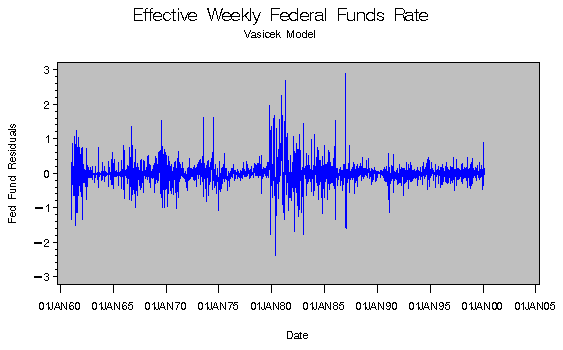Figure 1.1: Heteroscedastic residuals

Modeling non-constant variance, or heteroscedasticity, improves the efficiency of estimates of the parameters associated with the mean of a series and provides insight into the volatility of a series. SAS/ETS software provides capability to do linear and nonlinear regression with heteroscedastic models using the AUTOREG and MODEL procedures, respectively. This example makes use of the MODEL procedure.

# Details

One of the key assumptions of regression analysis is that the variance of the errors is constant across observations. This assumption is often violated when modeling time series or panel data, resulting in inefficient parameter estimates and inaccurate forecast error variance. Consider the following general model:
where
For models that are homoscedastic, h(t)=1. If you have a model which is heteroscedastic with known form, you can improve the efficiency of the estimates by performing a weighted regression. The weight variable, using this notation, is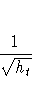If the errors for a model are heteroscedastic and the functional form of the variance is known, the model for the variance can be estimated along with the regression function.

To specify a functional form for the variance, assign the function to an H.var variable where var is the equation variable. For example, if you want to estimate the scale parameter for the variance of a simple OLS regression model

  y = a + b * x


you can specify

  proc model data=s;
y = a + b * x;
h.y = sigma**2;
fit y;


The above model has a constant variance, sigma**2. PROC MODEL estimates the constant-variance model by default, therefore the h.y equation is unnecessary in this case.

Now, consider the same model with the following functional form for the variance: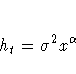This model is written as

  proc model data=s;
y = a + b * x;
h.y = sigma**2 * x**alpha;
fit y;


In addition to estimating the parameters, a and b, the MODEL procedure also estimates the parameters sigma and alpha, which are associated with the error variance.

## The Federal Funds Rate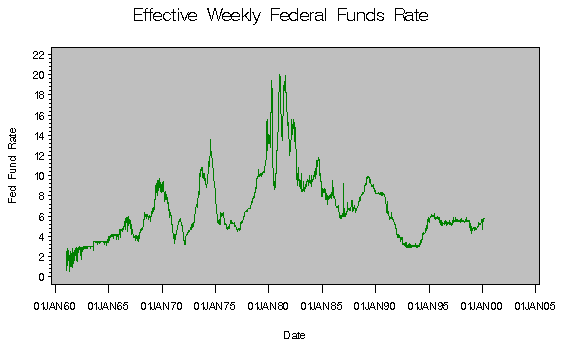Figure 1.2: The Weekly Federal Funds Rate

The following example uses weekly effective Federal funds data created from an average of daily figures from January 1961 to March 2000. A simple, commonly used rate model is the Vasicek model:
The following PROC MODEL statements are used to fit this model:

  proc model data=weeklyrates;
ffrate = lag(ffrate) + kappa  * (theta - lag(ffrate));
lag_ffrate = lag( ffrate );
label kappa = "Speed of Mean Reversion";
label theta = "Long term Mean";
fit ffrate / fiml breusch=( lag_ffrate ) out=resid outresid;
run;


The test for heteroscedasticity is positive and the residuals are shown in Figure 1.1. Note that the residuals are clearly heteroscedastic.

To correct for the heteroscedasticity, you can add a variance model. Another popular model for this type of data is the Cox Ingersoll Ross model, which accounts for the heteroscedasticity:
The following PROC MODEL statements are used to fit this model:

  proc model data=weeklyrates;
ffrate = lag(ffrate) + kappa * (theta - lag(ffrate));
h.ffrate = sigma**2 * lag(ffrate);
label kappa = "Speed of Mean Reversion";
label theta = "Long term Mean";
label sigma ="Constant part of variance";
fit ffrate / fiml out=resid outresid;
run;


The residuals are shown in Figure 1.3. The dependency on the lag of FFRATE has been removed, but the residuals remain heteroscedastic.

A GARCH(1,1) model is now considered: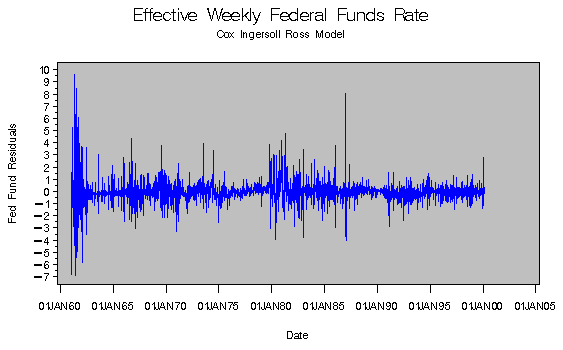Figure 1.3: Residuals from Cox Ingersoll Ross Model

The following PROC MODEL statements fit a GARCH(1,1) model:

  proc model data=weeklyrates;
ffrate = lag(ffrate) + kappa * (theta - lag(ffrate));
if ( _OBS_ = 1 ) then
h.ffrate = arch0 + arch1 * mse.ffrate + garch1 * mse.ffrate;
else
h.ffrate = arch0 + arch1 * zlag(resid.ffrate**2)
+ garch1 * zlag(h.ffrate);
fit ffrate / fiml out=resid outresid;
run;


The residuals from this estimation are shown in Figure 1.4. They look more homoscedastic than those produced by the other models, however, some additional investigation may be necessary on the data point occurring around 1987.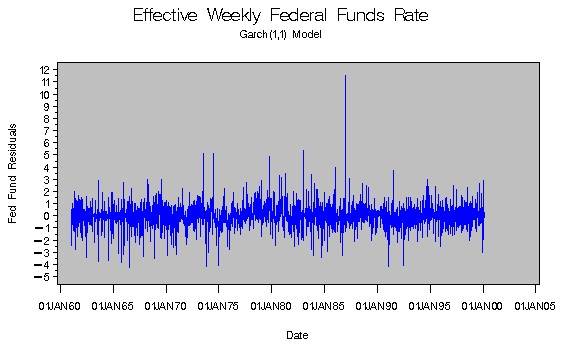Figure 1.4: Residuals from Garch(1,1)

# References

Chan, K.C. and Karolyi, G. Andrew and Longstaff, Francis A. and Sanders, Anthony B. (1992),"An Empirical Comparison of Alternate Models of the Short-Term Interest Rate", The Journal of Finance, 47(3), pp. 1209--1227.

Greene, William H. (1993), "Econometric Analysis," New York: Macmillian Publishing Company Inc.

SAS Institute Inc. (1993), SAS/ETS User's Guide, Version 8, Cary, NC: SAS Institute Inc.